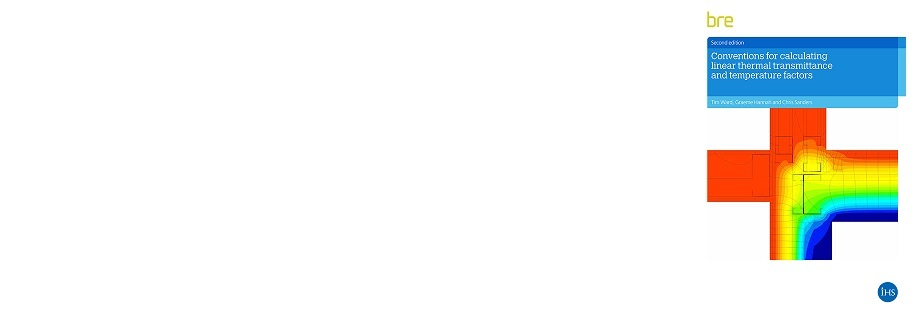•# BR497 (2016) - Clarifications and Notes

## Updated 27/10/16

Amendment No. 1 to BR497 2nd Edition 2016

BR497 Errata:

1.   At Page 41 Table B5 the value of Rsi given for downward heat flow is incorrect.  The value of 0.10 m²K/W should be 0.17 m²K/W.  This is also the case at Page 42 Table B6 and at Page 44 Table B7.

Notes & clarifications:

1.   At Page 44 Step 4 the equation is not as given on Page 23 for a suspended floor.

For consistency, the equation used here in Example 7 should have been the same as given on Page 23.  Note that either equation will provide the same result for psi‑value since the heat flow to be subtracted from the total heat flow will calculate to be the same.